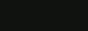NAME
SYNOPSIS
DESCRIPTION
USAGE
BUILT-IN FUNCTIONS
BUILT-IN VARIABLES
Source Code

## NAME

haskalc - a calculator written in the Haskell programming language

## DESCRIPTION

haskalc is a simple calculator written in the Haskell programming language.

## USAGE

The calculator currently supports integers and floating-point numbers and these basic operators:

- (subtraction)
* (multiplication)
/ (division)
% (modulo)
^ (exponentiation)
< (less than)
> (greater than)
= (assignment)

Usage:

x = 10 (variable)
f(x) = 2 * x (function)

Note: built-in variables and function cannot be overwritten.

?: (conditional)

Usage:

condition ? true_case : false_case

To exit the calculator write exit or send an EOF-character with Ctrl+D.

## BUILT-IN FUNCTIONS

sin(x)   - compute the sine of x
cos
(x)   - compute the cosine of x
tan
(x)   - compute the tangent of x
asin
(x)   - compute the arc sine of x
acos
(x)   - compute the arc cosine of x
atan
(x)   - compute the arc tangent of x
sinh
(x)   - compute the hyperbolic sine of x
cosh
(x)   - compute the hyperbolic cosine of x
tanh
(x)   - compute the hyperbolic tangent of x
asinh
(x)   - compute the area hyperbolic sine of x
acosh
(x)   - compute the area hyperbolic cosine of x
atanh
(x)   - compute the area hyperbolic tangent of x
sqrt
(x)   - compute the square root of x
log
(x)   - compute the natural logarithm of x
log10
(x)   - compute the common (base-10) logarithm of x
log2
(x)   - compute the binary (base-2) logarithm of x
ceil
(x)   - round x towards positive infinity
floor
(x)   - round x twoards negative infinity
truncate
(x)  - round x towards 0
round
(x)   - round x to the nearest integer value
gcd
(x, y)   - compute the greatest common divisor of x and y
lcm
(x, y)   - compute the least common multiple of x and y
toInt
(x)   - convert x to an integer.
toFloat
(x)   - convert x to a floating-point number.

## BUILT-IN VARIABLES

true   - boolean value of true.
false
- boolean value of false.
pi
- the constant pi.